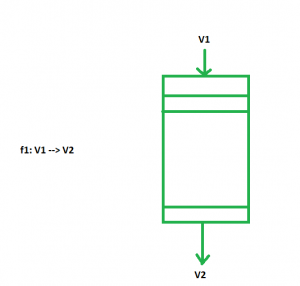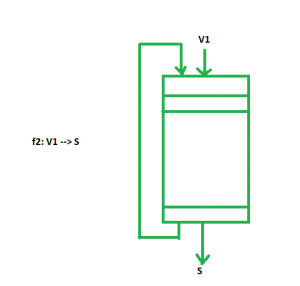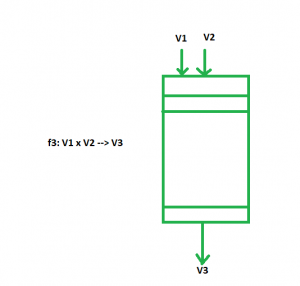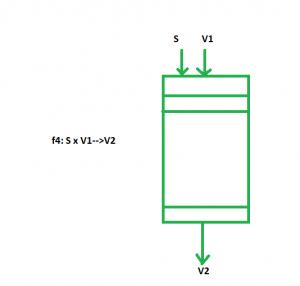# Vector instruction types

A Vector operand contains an ordered set of n elements, where n is called the length of the vector. All elements in a vector are same type scalar quantities, which may be a floating point number, an integer, a logical value, or a character.

Four primitive types of vector instructions are:

```f1 : V --> V
f2 : V --> S
f3 : V x V --> V
f4 : V x S --> V ```

Where V and S denotes a vector operand and a scalar operand, respectively.

The instructions, f1 and f2 are unary operations and f3 and f4 are binary operations.
The VCOM (vector complement), which complements each complement of the vector, is an f1 operation. The pipe lined implementation of f1 operation is shown in the figure:The VMAX (vector maximum), which finds the maximum scalar quantity from all the complements in the vector, is an f2 operation. The pipe lined implementation of f2 operation is shown in the figure:The VMPL (vector multiply), which multiply the respective scalar components of two vector operands and produces another product vector, is an f3 operation. The pipe lined implementation of f3 operation is shown in the figure:The SVP (scalar vector product), which multiply one constant value to each component of the vector, is f4 operation. The pipe lined implementation of f4 operation is shown in the figure:The Inputs are given as scalar components in the pipeline. Apart from these basic types of instructions, some special instructions may be used to facilitate the manipulation of vector data.

Attention reader! Don’t stop learning now. Get hold of all the important DSA concepts with the DSA Self Paced Course at a student-friendly price and become industry ready.

My Personal Notes arrow_drop_upCheck out this Author's contributed articles.

If you like GeeksforGeeks and would like to contribute, you can also write an article using contribute.geeksforgeeks.org or mail your article to contribute@geeksforgeeks.org. See your article appearing on the GeeksforGeeks main page and help other Geeks.

Please Improve this article if you find anything incorrect by clicking on the "Improve Article" button below.